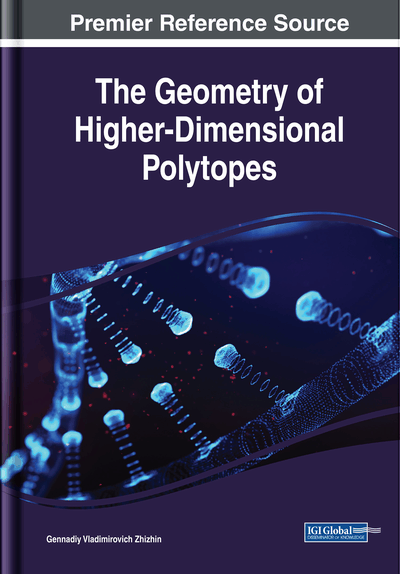# The Partition of n – Dimensional Space of Polytopic Prismahedrons

DOI: 10.4018/978-1-5225-6968-8.ch009
Available
\$37.50
No Current Special Offers

## Abstract

It is proved that polytopic prismahedrons have the necessary properties for partitioning the n - dimensional spaces of a face into a face, that is, they satisfy the conditions for solving the eighteenth Hilbert problem of the construction of n - dimensional spaces from congruent figures. General principles and an analytical method for constructing n -dimensional spaces with the help of polytopic prismahedrons are developed. On the example of specific types of the polytopic prismahedrons (tetrahedral prism, triangular prismahedron), the possibility of such constructions is analytically proved. It was found that neighboring polytopic prismahedrons in these constructions can have common geometric elements of any dimension less than n or do not have common elements.
Chapter Preview
Top

## Introduction

The problem of completing space by polyhedrons is one of the fundamental problems of mathematics, which has long attracted the attention of scientists. In 1900, D. Gilbert formulated 23 mathematical problems that require solution (Gilbert, 1901). One of these problems (eighteenth) was devoted to this question. It was formulated as follows: “Construction of space from congruent polyhedrons.” This problem is especially complicated in the case of n -dimensional spaces (Delone, 1969), and up to the end it has not been solved to this day under these conditions. In 1961, Delone (Delone, 1961) proved that if we require that polytopes in n - dimensional space be adjacent along entire (n -1) faces, then for any n there are only finitely many topologically different partitions of space into polytopes. These partitions are called normal. Delone and Sandakova (Delone & Sandakova, 1961) obtained a finite algorithm, in principle (according to the authors), which allows one to find all such partitions for a given n. In this case, the polytopes themselves (stereohedrons by the Delone terminus) of these partitions for a fixed n can be only of a finite number of topologically different types. However, unfortunately, the authors did not obtain concrete examples of normal partitions of higher - dimensional space into polytopes and the specific types of corresponding polytopes. The question of the existence of fundamental domains in n - dimensional space is quite nontrivial. The main definition of the fundamental domain it was formulated by Delone (Delone, 1969). The fundamental domain of a group of motions is a set of points of space such that, firstly, all its points are not equivalent to each other with respect to the group of motions, and secondly, any point of space is equivalent to some point of this region relative to the group of motions. What kind of these fundamental areas for a space with a dimension greater than three remains to this day is unknown. Here we must also keep in mind the existence of polytopes, whose congruential copies can fill a gapless space, but each of them is not a fundamental area (Reingard, 1928).

The partitions of higher dimensional space first it were given by Zhizhin (Zhizhin, 2017). He showed that polytopes of partitions of higher - dimensional space are the polytopic prismahedrons. Moreover, the normality condition in these partitions is not obligatory, i.e. in the n - dimensional space there are polytopes adjacent along entire (n -1) faces, but there are polytopes adjacent along the faces of another dimension.

One begin our consideration of the problem of partitioning a space of higher dimension from the reduction of concrete examples of these partitions.

## Key Terms in this Chapter

New Paradigm of Discrete n – Dimension World: The elementary cells of the translational filling of the n -dimensional space are the polytopic prismahedrons - the stereohedrons, of which Delone spoke, but he did not give a single concrete example of stereohedron. Polytopic prismahedrons, filling the n -dimensional space, as shown by direct construction, can have common elements in the entire range of dimensions up to the dimension of the facets, or do not have any common elements. In addition to the translational filling of the n -dimensional space, there is a hierarchical filling of the space, which is inextricably linked with the scaling process, i.e. discrete scale change of the figure. Translational filling of space can be combined with hierarchical filling of space. In this case, in principle, in each point of space there is an asymptotic decrease or increase in the scale of the figure (as an expansion of the Universe from each of its points). Delone's provisions on an “empty” ball, the finite minimum and maximum distances between the points of a discrete system, are not used. Thus, Hilbert's problem acquires a completely new content.

The Congruent Polyhedrons: Polyhedrons that are compatible with motion.

The 5 – Simplex - Prism: The product of a 4 - simplex by a segment.

The Polytopic Prismahedron: The product of polytopes.

The Triangular Prismahedron: The product of a triangle by a triangle.

The Tetrahedral Prism: The product of a tetrahedron by a segment.

## Complete Chapter List

Search this Book:
Reset